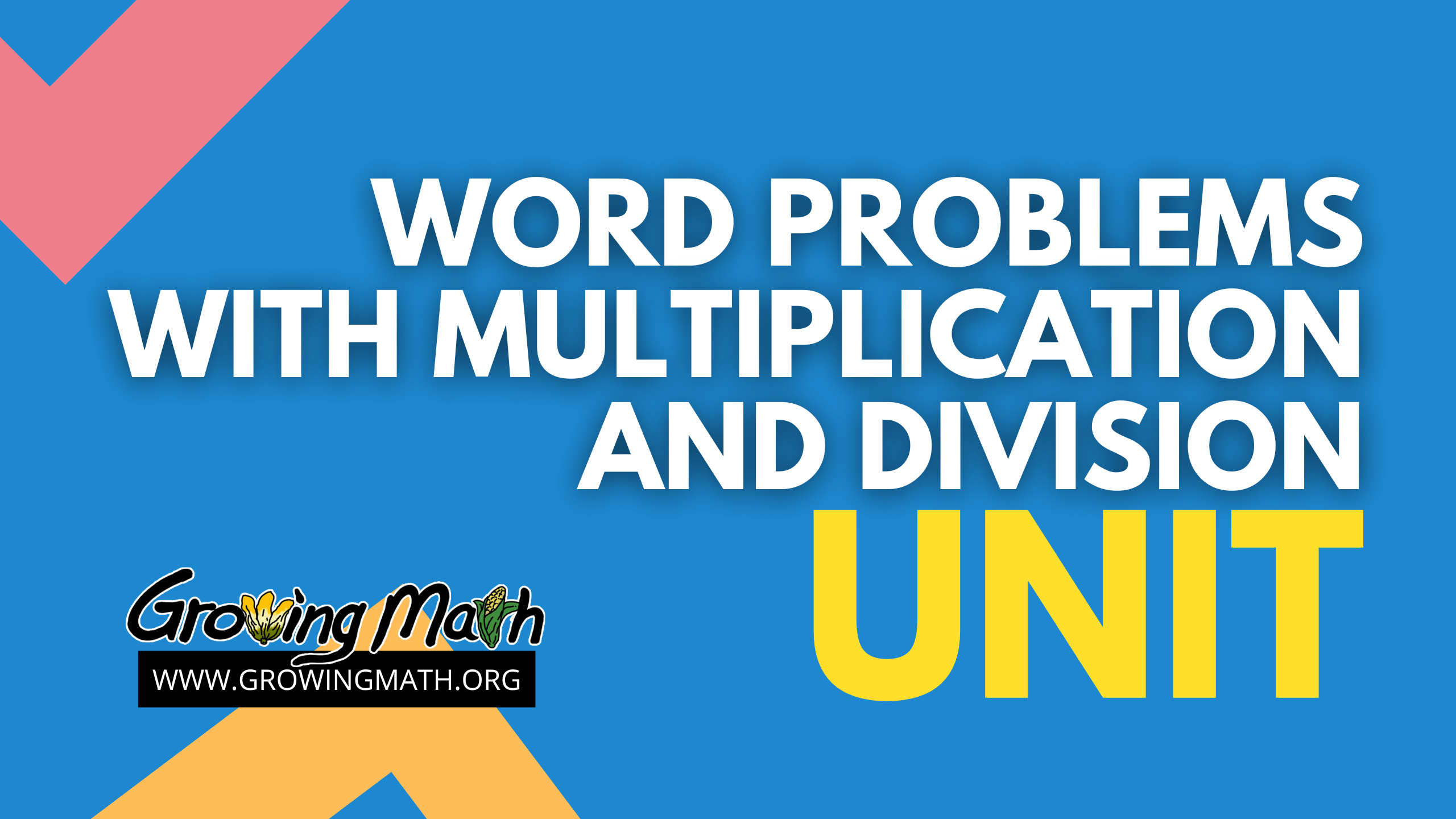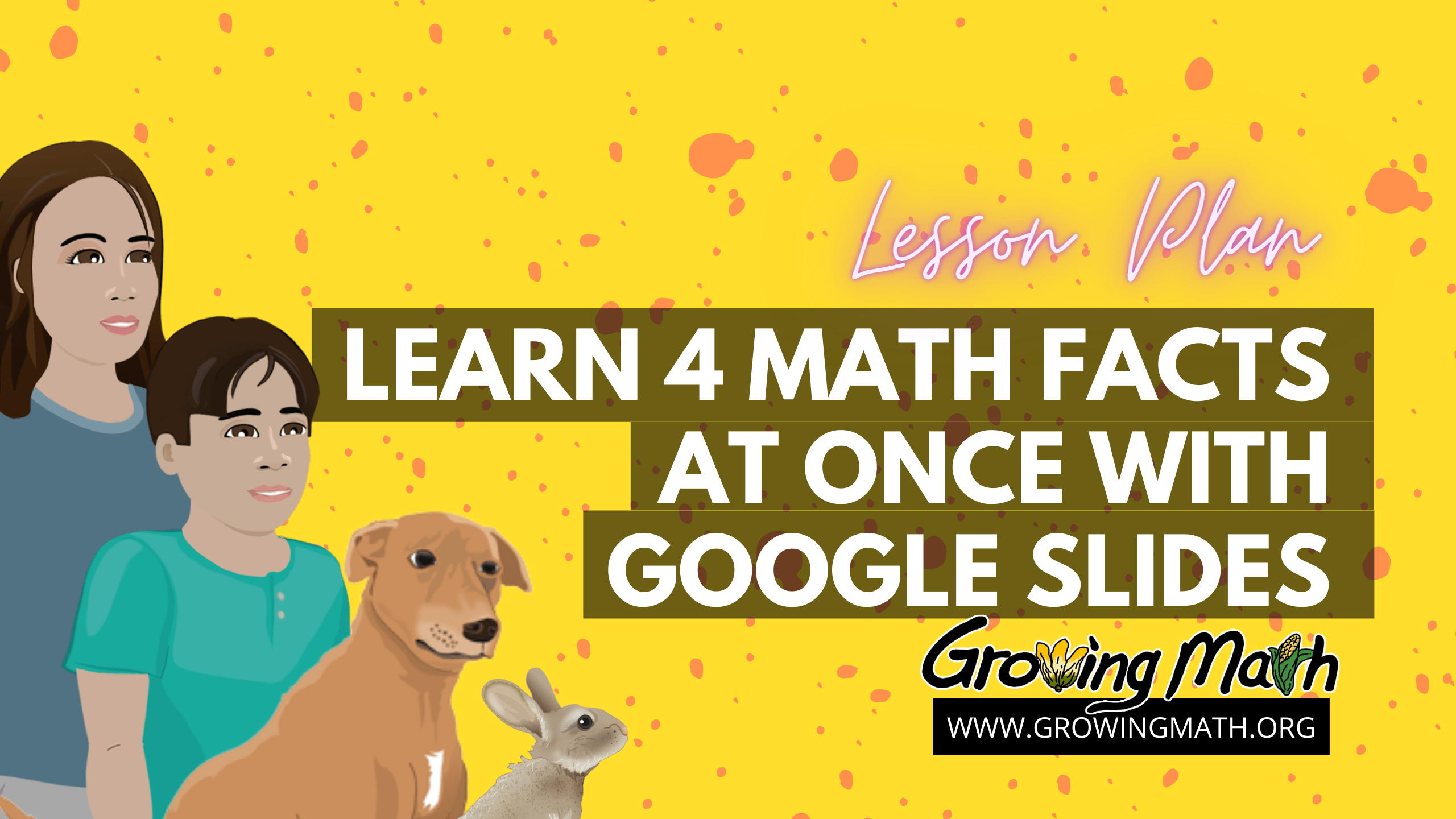# Category Archives: division# UNIT: Word Problems with Multiplication and Division

## Standards

CCSS.MATH.CONTENT.3.MD.D.8 Solve real world and mathematical problems involving perimeters of polygons, including finding the perimeter given the side lengths, finding an unknown side length, and exhibiting rectangles with the same perimeter and different areas or with the same area and different perimeters.
CCSS.Math.Content.3.OA.B.5 Apply properties of operations as strategies to multiply and divide.
CCSS.MATH.CONTENT.3.OA.D.8 Solve two-step word problems using the four operations. Represent these problems using equations with a letter standing for the unknown quantity. Assess the reasonableness of answers using mental computation and estimation strategies including rounding.
CCSS.MATH.CONTENT.4.MD.A.3 Apply the area and perimeter formulas for rectangles in real world and mathematical problems.
CCSS.MATH.CONTENT.4.NBT.B.4 Fluently add and subtract multi-digit whole numbers using the standard algorithm

Five hours

## Technology Required

If teaching in person, the teacher will need a computer and projector or smart board to show the videos, or students can be given the links to watch on their own devices. Students will need a PC, Mac or Chromebook or tablet. Making Camp Premium, Making Camp Lakota and Making Dakota are all playable on any web browser on those devices. Spirit Lake: The Game, playable on Mac or Windows computers also teaches these same concepts or students can watch the videos from the game on any device with a browser.

## UNIT SUMMARY

This cross-curricular unit teaches solving word problems with multiplication and division with a variety of strategies and in contexts ranging from agriculture to traditional dwellings.

## Lessons

Lesson Multiplying to find perimeter of polygons

In this 45-minute lesson, students will learn how to compute perimeter of different polygons using multiplication and apply those skills in game-based practice problems. They will then learn about different Indigenous traditional dwellings. The lesson ends with students contributing to and solving problems that integrate the reading on dwellings and perimeter in an online assessment.

Multiplication word problems

In this 40-minute integrated lesson, students learn about responsibilities of children in traditional Dakota society and discuss their responsibilities today. They learn a problem-solving strategy that can be applied to a wide range of situations, including mathematics. Students play Spirit Lake: The Game or watch videos solving multiplication problems set in the context of a story based on Dakota culture.

Travois, multiplication and 2-step problems

In this 45-minute lesson, the students will develop an understanding of the meanings of the four operations of whole numbers through activities and problems involving real life scenarios from Indigenous history. Students use properties of operations to calculate products of whole numbers, using increasingly sophisticated strategies based on these properties to solve using the four operations problems involving single-digit factors. It includes educational videos, games and video presentations that can be used for reviews and daily practice.

Problem-solving with pigs: Start at the end

This 75-minute cross-curricular lesson includes activities and instruction in agricultural science and math. Students begin by watching a video and learning about pig farms. After making their own pig barn, they watch two short videos about solving math problems. This information is then applied to solve multiplication and division word problems during a presentation on math around the pig farm. Students end playing one of the Making Camp games to reinforce skills and knowledge.

Lesson. Problem-Solving Two ways

his 40-minute lesson assumes that students have some familiarity with multiplication of one-digit numbers and division with one-digit divisors. Students are introduced to the various means of problem solving in a brief presentation. They watch a video on visualization, then solve a problem that asks them to visualize. After watching a video on building a model, students build and/or draw their own model of a multiplication problem or property. Lesson concludes with game play to reinforce these problem-solving strategies and learn more. T

Learn 4 Math Facts at Once with Google Slides

This 60-minute lesson starts with a video as an ice breaker. Then, students read or listen to a presentation explaining how each math fact is actually four. Students complete an activity where they create their own math facts slides. A recommended video explains features of Google slides. Students complete the lesson playing Making Camp Dakota, solving word problems using division.# Learn 4 Math Facts at Once with Google Slides

## Standard

CCSS.MATH.CONTENT.3.OA.A.1 Interpret products of whole numbers, e.g., interpret 5 × 7 as the total number of objects in 5 groups of 7 objects each.

CCSS.MATH.CONTENT.3.OA.A.2 Interpret whole-number quotients of whole numbers, e.g., interpret 56 ÷ 8 as the number of objects in each share when 56 objects are partitioned equally into 8 shares.

CCSS.MATH.CONTENT.4.NBT.B.4
Fluently add and subtract multi-digit whole numbers using the standard algorithm.

45-60 minutes

## Summary

Start with a video as an ice breaker. Then, students read or listen to a presentation explaining how each math fact is actually four. Students complete an activity where they create their own math facts slides. A recommended video explains features of Google slides. Students complete the lesson playing Making Camp Dakota, solving word problems using division.

## Listen to/ Read a presentation on four math facts at a time

This presentation explains how one math fact is actually four because 7 x 6 = 42 means that 42 ÷ 7 = 6 and also that 6 x 7 = 42 and 42 ÷ 6 = 7. As an added bonus, it includes some ranch vocabulary like cattle, steer, heifer and bull.

After the teacher has given the first part of the presentation, students are challenged to first show how a given math fact is actually four math facts, using steers. Next, students create their own examples. The easiest way to do this is through assignment in Google classroom or other system, including email, giving students their own copies of the slides to modify.

### Recommended Video: Math Facts with Google Slides

If your students are unsure how to copy and paste, how to select multiple elements at a time, rotate objects or insert a duplicate slide, it is all in this video. Why not watch the video first? Because students often pay more attention once they realize they have questions that can be assigned by the assigned video.

### Play Making Camp Dakota

Play Making Camp Dakota: Past and Present to learn about Dakota history and culture and solve division problems, such as dividing the people on the buffalo hunt. Students can be assigned to go directly to the game here or to access it by selection in the games portal for kids.

## Assessment

Two types of assessment are included in this lesson:

1. Students create their own math facts slides, and in-game assessment.
2. Problems are scored automatically in Making Camp Dakota and data are available through the teacher reports.

## Differentiated Instruction

Many students with learning disabilities have stronger achievement in one area than another, for example their grade level in reading is higher than in mathematics. For these students in higher grades, an assignment to learn to use presentation software such as Google slides is age appropriate and intrinsically interesting and at the same time increases their knowledge of basic math facts.# Buffalo Hunts and Division

## 📖 Standard

CCSS.MATH.CONTENT.5.NBT.B.5 Fluently multiply multi-digit whole numbers using the standard algorithm.

CCSS.MATH.CONTENT.5.NBT.A.3.A Read and write decimals to thousandths

CCSS.MATH.CONTENT.5.NBT.A.4 Use place value understanding to round decimals to any place.

NOTE: Teachers who have not yet covered decimals in their class have an option within the lesson to only teach the first standard.

25- 30 minutes

## 📲 Technology Required

Computer or mobile device

## 📃 Summary

Begin with a video on long division (optional) or a presentation on uses of division from the playground to the buffalo hunt. Watch a short video working long division problems. Finish with practicing long division in Making Camp Dakota. Short videos on Dakota buffalo hunt traditions and related math lessons are also linked.

## 📚Lesson

### Video: Optional for Review

If your students need a review of the steps in long division, we recommend this six-minute video that uses the acronym HMS ↓ to teach students to :

• Multiply
• Subtract
• Bring down

### Presentation on Division and Buffalo Hunts

This Google slides presentation gives common factors in buffalo hunts across different tribes and explains how whether it is computing the number of hides a horse can carry or how long each group gets to play at recess, division is always useful.

NOTE: This presentation includes two slides that reference converting a fraction to a decimal. If you have not yet introduced this concept, those slides can be deleted.

### Play a Game – Making Camp Dakota

Select the Buffalo Icon (top left corner) in the first MATH screen to practice long division.

### Related Lessons

For more instruction on division check out these two lessons:

### Related videos

Dakota deer and buffalo hunting# Breaking Down Division with Remainders

Lesson by James Gumela

## 📖 STANDARD

CCSS.MATH.CONTENT.4.NBT.6 Find whole-number quotients and remainders with up to four-digit dividends and one-digit divisors, using strategies based on place value, the properties of operations, and/or the relationship between multiplication and division. Illustrate and explain the calculation by using equations, rectangular arrays, and/or area models.

30-45 minutes

## 📃 SUMMARY

This lesson plan will explore how students interpret remainders in the context of a problem. They will learn how to divide and have a number leftover. Students will be using the concept of division and remainders. An instructional video will be presented as well as a PowerPoint presentation and an educational game that can be used to reinforce the concept of remainders with assessment data.

## 📚 Lesson

### Watch Video

In division, remainders occur when a number can’t be evenly divided. This video will help the students understand the concept of remainders.

Also available in spanish:

### Break Down Long Division in a Presentation

This Google slides presentation: Division with remainders reviews the parts of a division problem; quotient, division and divisor, then works a sample problem step by step. The presentation ends with a practical question in a word problem- when you have a remainder, what do you do with the amount that remains?

### Play a Game – Making Camp Dakota

Making Camp Dakota follows a family at a pow-wow as the children learn about Dakota culture through stories from their elders and apply their long division skills along the way.